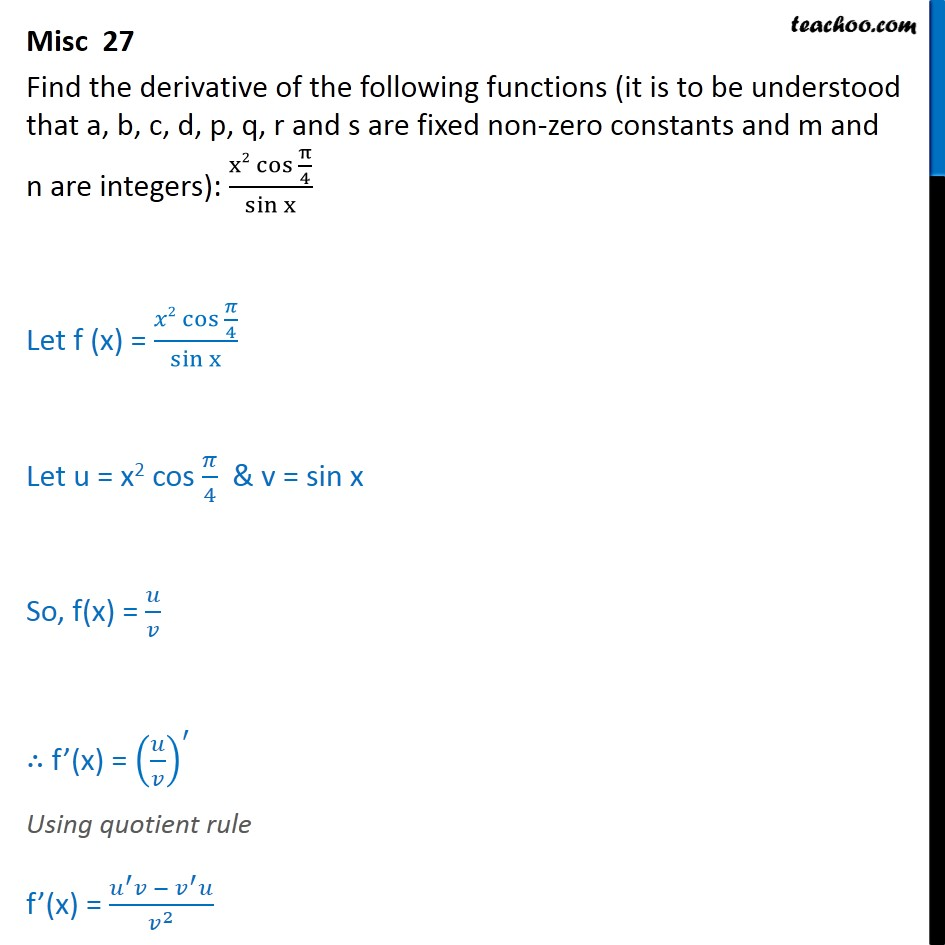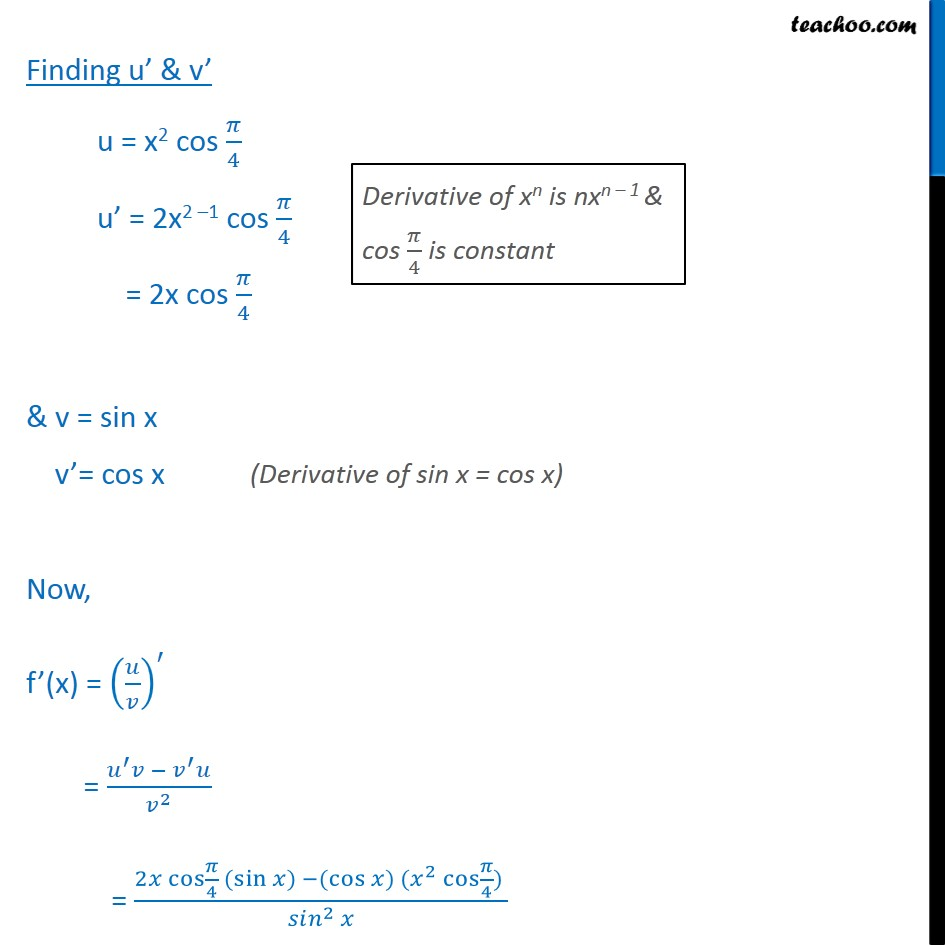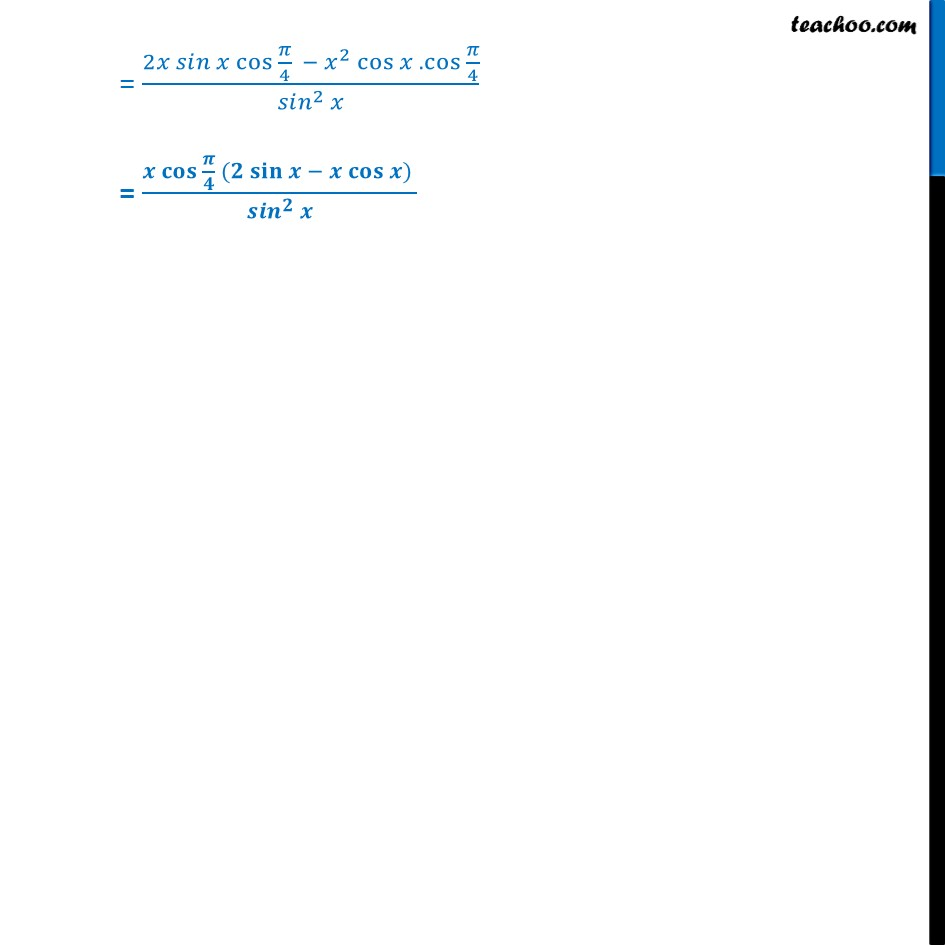1. Class 11
2. Important Question for exams Class 11
3. Chapter 13 Class 11 Limits and Derivatives

Transcript

Misc 27 Find the derivative of the following functions (it is to be understood that a, b, c, d, p, q, r and s are fixed non-zero constants and m and n are integers): x2 cos ﷮ π﷮4﷯﷯﷮ sin﷮x﷯﷯ Let f (x) = 𝑥2 cos ﷮ 𝜋﷮4﷯﷯﷮sin x﷯ Let u = x2 cos 𝜋﷮4﷯ & v = sin x So, f(x) = 𝑢﷮𝑣﷯ ∴ f’(x) = 𝑢﷮𝑣﷯﷯﷮′﷯ Using quotient rule f’(x) = 𝑢﷮′﷯𝑣 − 𝑣﷮′﷯𝑢﷮ 𝑣﷮2﷯﷯ Finding u’ & v’ u = x2 cos 𝜋﷮4﷯ u’ = 2x2 –1 cos 𝜋﷮4﷯ = 2x cos 𝜋﷮4﷯ & v = sin x v’= cos x Now, f’(x) = 𝑢﷮𝑣﷯﷯﷮′﷯ = 𝑢﷮′﷯𝑣 − 𝑣﷮′﷯𝑢﷮ 𝑣﷮2﷯﷯ = 2𝑥 cos﷮ 𝜋﷮4﷯﷯ ( sin﷮𝑥)﷯ −( cos﷮𝑥) ( 𝑥﷮2﷯ cos﷮ 𝜋﷮4﷯) ﷯﷯﷮ 𝑠𝑖𝑛﷮2﷯ 𝑥﷯ = 2𝑥 𝑠𝑖𝑛 𝑥 cos ﷮ 𝜋﷮4﷯﷯ − 𝑥﷮2﷯ cos﷮𝑥 . cos ﷮ 𝜋﷮4﷯﷯﷯﷮ 𝑠𝑖𝑛﷮2﷯ 𝑥﷯ = 𝒙 𝐜𝐨𝐬 ﷮ 𝝅﷮𝟒﷯﷯ (𝟐 𝐬𝐢𝐧﷮𝒙 − 𝒙 𝐜𝐨𝐬﷮𝒙) ﷯﷯﷮ 𝒔𝒊𝒏﷮𝟐﷯ 𝒙﷯

Chapter 13 Class 11 Limits and Derivatives

Class 11
Important Question for exams Class 11